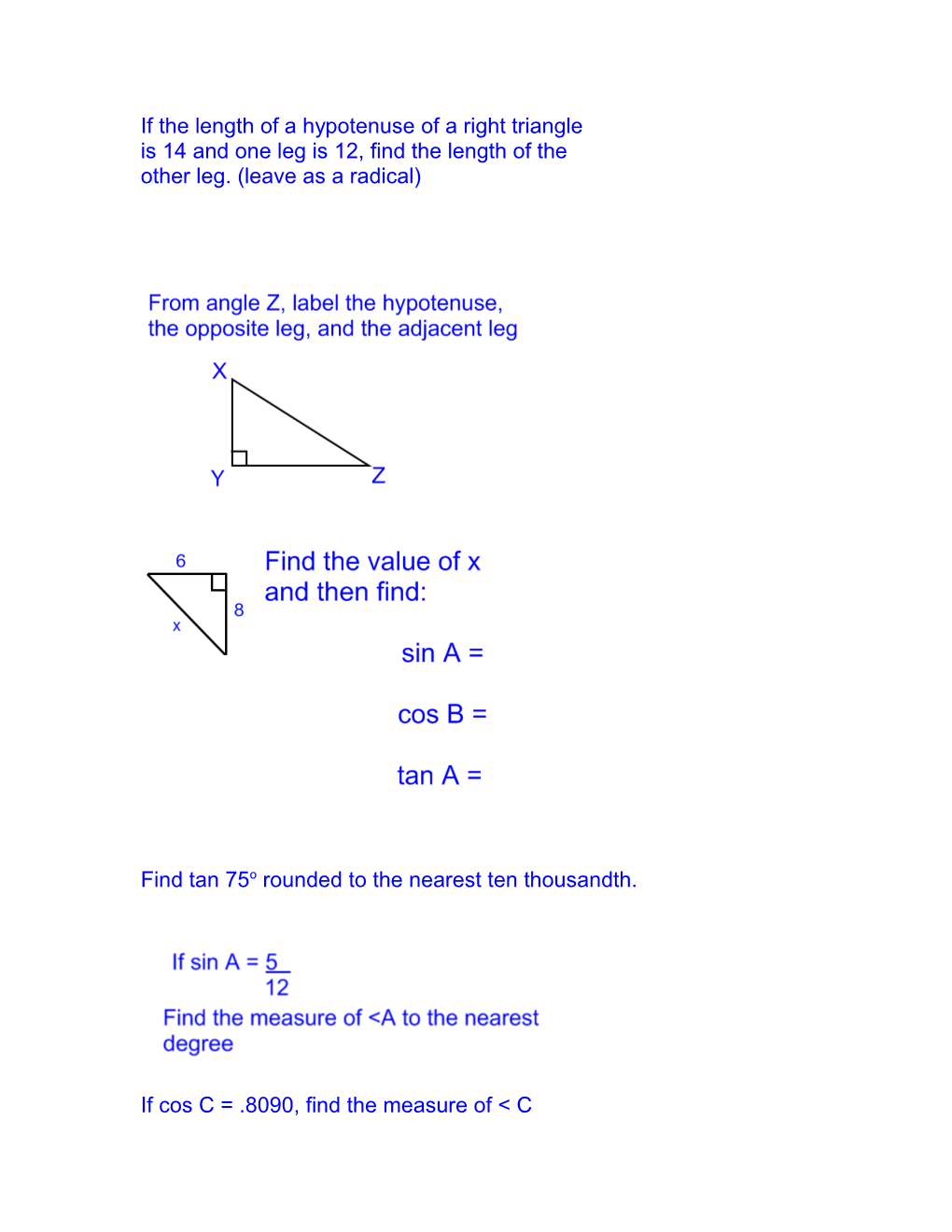# If the Length of a Hypotenuse of a Right TriangleIf the length of a hypotenuse of a right triangle

is 14 and one leg is 12, find the length of the

other leg. (leave as a radical)Find tan 75o rounded to the nearest ten thousandth.

If cos C = .8090, find the measure of < C

The angle of elevation of the top of a building from a point on the ground 150 feet from the base if the building is 50°, Find

the height of the building to the nearest foot.

From the top of a lighthouse 80 feet high, the angle of depression of a boat out at sea is 28°. Find, to the nearest foot, the distance from the boat to the base of the lighthouse, the base being at sea level.

A wooden beam 6 meters long leans against a wall and makes an angle of 71 degrees with the ground. Find to the nearest tenth of a meter how high up the wall the beam reaches.

A ladder that leans against a building makes an angle of 75 degrees with the ground and reaches a point on the building 9.7 meters above the ground. Find to the nearest meter the length of the ladder.

A 25 foot ladder leans against a building and makes an angle of 72 degrees with the ground. Find to the nearest foot the distance between the foot of the ladder and the building.

An airplane rises at an angle of 14 degrees with the ground. Find to the nearest hundred feet the distance the airplane has flown when it has covered a horizontal distance of 1,500 feet.

A ladder 13 feet long leans against a building and reaches the ledge of a window. If the foot of the ladder is 5 feet from the foot of the building, how high is the window ledge above the ground to the nearest foot?

Mrs. Dombrowski traveled 18 kilometers north and then 20 kilometers east. In a straight line, how far was she from her starting point?In right triangle CAN, the length of the hypotenuse CA is 72 meters and the measure of angle C is 26 degrees.

a.) Find CN and AN to the nearest meter.

b.) Use the Pythagorean Theorem to show that the results obtained in part a(before rounding) are correct.

Angles T and K are two acute angles of a right triangle. If the Tan T is 15/4.

a.) What is that ratio of the Tan K?

b.)What is the relationship between these ratios?

In all isosceles right triangles, the tangent of either acute angle is always the same ratio. What is the ratio for the tangent of the acute angle of an isosceles triangle?